Open in App
Not now

# James A. Garfield’s Proof of the Pythagorean Theorem

• Last Updated : 24 Feb, 2022

Pythagorean theorem is a theorem for right-angled triangles, it is also referred to as the Pythagoras theorem. It is used to show the connection in the sides of a triangle which is a right-angled triangle. According to this theorem sum of squares of any two small sides is equal to the square of the biggest side. The small sides of a right-angled triangle are perpendicular and base while the biggest side is known as hypotenuse.

The discovery of this theorem is linked with an ancient Greek philosopher who was Pythagoras and hence it is called Pythagoras Theorem.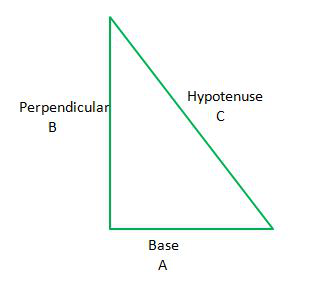Expression for Pythagoras Theorem:

(Base)2 + (Perpendicular)2   =    (Hypotenuse)2

A2       +         B2                  =         C2

### President James A. Garfield’s proof of the Pythagorean theorem

This proof of the Pythagorean Theorem was given by President James A. Garfield’s, who was the 20th president and was elected in the year 1881, he really likes maths and gave this proof of the Pythagorean theorem.

Let’s prove the theorem

Step 1: Draw a right-angled triangle with sides A, B and C.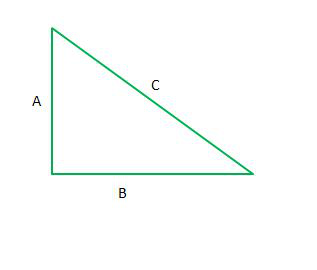Step 2: Draw another triangle of the same measurement, but side A of the first triangle should form a straight line with side B of the second triangle.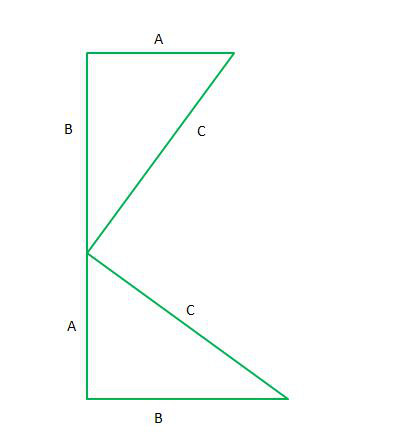Step 3: Now mark the angles in both the triangles, let’s take one angle as θ so the other angle will be 90 – θ as it is a right-angled triangle and the third angle is 90 degrees, and the sum of all angles in a triangle is 180 degrees.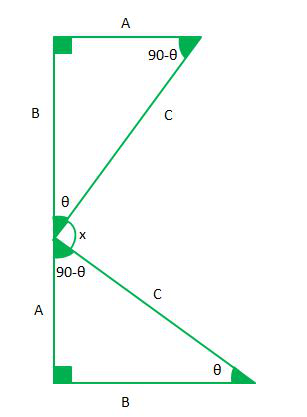Step 4: Now we know that sum of angles on a straight line is 180 degrees so using this we will find angle x.

θ +  x + 90 – θ = 180°

x + 90 = 180°

x = 90°

Hence, x is 90° angle.

Step 5: Now draw a straight line to make a third triangle with two sides as C and C and mark the triangles as 1, 2 and 3.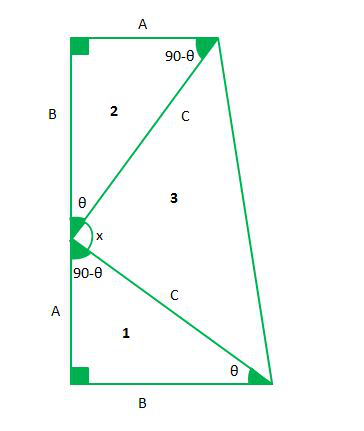Step 6: Now the whole figure resembles a trapezium so we will find its area.

Area of Trapezium = 1/2 × (Sum of parallel sides) × ( Height)

In the above figure, parallel sides are A and B and the height of the trapezium is A+B.

Area = 1/2(A+B)×(A+B)

Step 7: The same area can also be calculated by adding the areas of 3 right-angled triangles.

• Area of a right-angled triangle = 1/2(Base)×(Height)
• Area of the whole figure = Area of first triangle + Area of second triangle + Area of the third triangle
• Area of whole figure = 1/2(A)×(B) + 1/2(A)×(B) + 1/2(C)×(C)
• Area of whole figure = A×B + C2/2

Step 8: Both areas should be equal so put them equal to one another.

1/2(A+B)×(A+B) = A×B + C2/2

(A+B)2 = 2(A×B) + C2

A2 + B2 + 2(A×B) = 2(A×B) + C2

A2 + B2 = C2

Hence we proved the Pythagorean theorem

### Sample Problems

Problem 1: Find the length of the hypotenuse of a right-angled triangle whose height is 31 cm and whose base is 480 cm?

Solution:

Using Pythagorean theorem, a2 + b2 = c2

So 312 + 4802 = c2

hence c = √(961 + 230400)

c = √231361

c = 481 cm

Length of hypotenuse = 481 cm

Problem 2: What are the area of a trapezium whose height is 10 cm and parallel sides are 8 and 12 cm long.

Solution:

Area of trapezium can be calculated using formula

Area of Trapezium = 1/2(sum of parallel sides)×(height)

= 1/2(8 + 12)×(10)

= 100

Area of trapezium is 100 cm2

Problem 3: What is the area of a right-angled triangle whose hypotenuse is 10 cm and base is 6 cm.

Solution:

To find the area of the right-angled triangle, we need base and height but we only have base.

So use the Pythagorean theorem to find the perpendicular

Perpendicular = √(hypotenuse)2 – (base)2

= √102 – 62

perpendicular = √100 – 36

perpendicular = √64

perpendicular = 8 cm

Now Area = 1/2(Base)×(Perpendicular)

= 1/2(6)×(8)

= 24

The area of the triangle is 24 cm2

Problem 4: Find the length of the hypotenuse of a right-angled triangle whose height is 69 cm and whose base is 260 cm?

Solution:

Using Pythagorean theorem, a2 + b2 = c2

So, 692 + 2602 = c2

hence c = √(4761 + 67600)

c = √72361

c = 269 cm

Length of hypotenuse = 269 cm

Problem 5: What is the area of a trapezium whose height is 20 cm and parallel sides are 5 and 18 cm long.

Solution:

Area of trapezium can be calculated using formula

Area of Trapezium = 1/2(sum of parallel sides)×(height)

= 1/2(5 + 18)×(20)

= 230

Area of trapezium is 230 cm2

My Personal Notes arrow_drop_up